# Difference between revisions of "Hausdorff space"

## Definition

### Equivalent definitions in tabular format

A topological space is said to be Hausdorff if it satisfies the following equivalent conditions:

No. Shorthand A topological space is termed Hausdorff if ... A topological space$X$ is termed Hausdorff if ...
1 Separation axiom given any two distinct points in the topological space, there are disjoint open sets containing the two points respectively. given any two points$x \ne y \in X$, there exist open subsets$U \ni x$ and$V \ni y$ such that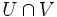$U \cap V$ is empty
2 Diagonal in square the diagonal is closed in the product of the space with itself in the product space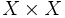$X \times X$, endowed with the product topology, the diagonal, viz., the subset given by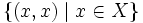$\{ (x,x) \mid x \in X \}$ is a closed subset
3 Ultrafilter convergence every ultrafilter of subsets converges to at most one point if$S_\alpha$ is an ultrafilter of subsets of$X$, then there is at most one$x \in X$ for which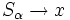$S_\alpha \to x$.
4 Separation axiom for finite subsets given any finite collection of distinct points the topological space, there are open subsets containing each of the points in that finite subset that have pairwise trivial intersection. for any finite collection$x_1, x_2, \dots, x_n$ of distinct points in$X$, there exists a finite collection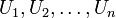$U_1, U_2, \dots, U_n$ of open subsets of$X$ such that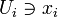$U_i \ni x_i$ for all$i$ and$U_i \cap U_j$ is empty for$i \ne j$.
5 Separation axiom using basis open subsets (choose a basis of open subsets for the topological space) given any two distinct points in the topological space, there are disjoint open sets from the basis containing the two points respectively. (choose a basis of open subsets for the topological space) given any two points$x \ne y \in X$, there exist open subsets$U \ni x$ and$V \ni y$ such that both$U$ and$V$ are in the basis and$U \cap V$ is empty
6 Separation axiom for finite subsets using basis open subsets (choose a basis of open subsets for the topological space) given any finite collection of distinct points the topological space, there are open subsets (all from the basis) containing each of the points in that finite subset that have pairwise trivial intersection. for any finite collection$x_1, x_2, \dots, x_n$ of distinct points in$X$, there exists a finite collection$U_1, U_2, \dots, U_n$ of open subsets of$X$ (all from the basis) such that$U_i \ni x_i$ for all$i$ and$U_i \cap U_j$ is empty for$i \ne j$.
7 Separation axiom for two compact subsets any two disjoint compact subsets can be separated by disjoint open subsets given any compact subsets$A_1,A_2$ of$X$ (i.e.,$A_1,A_2$ are both compact spaces in the subspace topology), there exist disjoint open subsets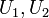$U_1,U_2$ such that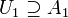$U_1 \supseteq A_1$ and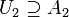$U_2\supseteq A_2$.
8 Separation axiom for finitely many compact subsets any finite collection of pairwise disjoint compact subsets can be separated by a finite collection of pairwise disjoint open subsets given a finite collection$A_1,A_2,\dots,A_n$ of pairwise disjoint compact subsets of$X$, there exist pairwise disjoint open subsets$U_1,U_2,\dots,U_n$ of$X$ such that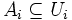$A_i \subseteq U_i$ for each$i$.
9 Separation axiom between point and compact subset given any point and any compact subset not contianing it, the point and the compact subset can be separated by disjoint open subsets. for any$x \in X$ and any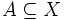$A \subseteq X$ such that$A$ is compact and$x \notin A$, there exist disjoint open subsets$U,V$ of$X$ such that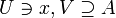$U \ni x, V \supseteq A$.
10 preregular and Kolmogorov it is both a preregular space and a Kolmogorov space (i.e., a$T_0$ space)

### Equivalence of definitions

Further information: equivalence of definitions of Hausdorff space

## Examples

### Extreme examples

• The empty space is a Hausdorff space. For this space, the Hausdorffness condition is vacuously satisfied.
• The one-point space is a Hausdorff space. For this space, the Hausdorffness condition is vacuously satisfied.
• Any discrete space (i.e., a topological space with the discrete topology) is a Hausdorff space.

### Non-examples

This article defines a property of topological space that is pivotal (viz important) among currently studied properties of topological spaces

In the T family (properties of topological spaces related to separation axioms), this is called: T2

View a complete list of basic definitions in topology

## Metaproperties

Metaproperty name Satisfied? Proof Statement with symbols
product-closed property of topological spaces Yes Hausdorffness is product-closed If$X_i, i \in I$ is a (finite or infinite) collection of Hausdorff topological spaces, the product of all the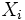$X_i$s, equipped with the product topology, is also Hausdorff.
box product-closed property of topological spaces Yes Hausdorffness is box product-closed If$X_i, i \in I$ is a (finite or infinite) collection of Hausdorff topological spaces, the product of all the$X_i$s, equipped with the box topology, is also Hausdorff.
subspace-hereditary property of topological spaces Yes Hausdorffness is hereditary Suppose$X$ is a Hausdorff space and$A$ is a subset of$X$. Under the subspace topology,$A$ is also Hausdorff.
refining-preserved property of topological spaces Yes Hausdorffness is refining-preserved Suppose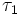$\tau_1$ and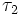$\tau_2$ are two topologies on a set$X$, such that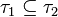$\tau_1 \subseteq \tau_2$, i.e., every subset of$X$ open with respect to$\tau_1$ is also open with respect to$\tau_2$. Then, if$X$ is Hausdorff with respect to$\tau_1$, it is also Hausdorff with respect to$\tau_2$.
local property of topological spaces No Hausdorffness is not local It is possible to have a topological space$X$ such that, for every$x \in X$, there exists an open subset$U \ni x$ that is Hausdorff but$X$ is not itself Hausdorff. Spaces with this property are called locally Hausdorff spaces.
closure-local property of topological spaces Yes Hausdorffness is closure-local If$X$ is a topological space such that, for every$x \in X$, there exists an open subset$U \ni x$ such that the closure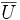$\overline{U}$ is Hausdorff, then$X$ is Hausdorff.

## Relation with other properties

### Stronger properties

Property Meaning Proof of implication Proof of strictness (reverse implication failure) Intermediate notions
normal Hausdorff space$T_1$ and any two disjoint closed subsets are separated by disjoint open subsets Hausdorff not implies normal Functionally Hausdorff space, Regular Hausdorff space, Tychonoff space, Urysohn space|FULL LIST, MORE INFO
collectionwise normal Hausdorff space$T_1$ and collectionwise normal: any discrete collection of closed subsets is separated by disjoint open subsets (via normal) (via normal) |FULL LIST, MORE INFO
regular Hausdorff space$T_1$ and any point and closed subset not containing it are separated by disjoint open subsets Hausdorff not implies regular Urysohn space|FULL LIST, MORE INFO
Tychonoff space$T_1$ and completely regular: any point and disjoint closed subset are separated by a continuous function to$[0,1]$ (via regular) (via regular) Functionally Hausdorff space, Regular Hausdorff space, Urysohn space|FULL LIST, MORE INFO
collectionwise Hausdorff space any discrete set of points can be separated by disjoint open subsets collectionwise Hausdorff implies Hausdorff Hausdorff not implies collectionwise Hausdorff |FULL LIST, MORE INFO
functionally Hausdorff space any two distinct points can be separated by a continuous function to the reals Urysohn space|FULL LIST, MORE INFO
Urysohn space any two distinct points can be separated by closure-disjoint open subsets |FULL LIST, MORE INFO
metrizable space underlying topology of a metric space metrizable implies Hausdorff Hausdorff not implies metrizable Collectionwise Hausdorff space, Completely regular space, Functionally Hausdorff space, Monotonically normal space, Moore space, Normal Hausdorff space, Regular Hausdorff space, Submetrizable space, Tychonoff space|FULL LIST, MORE INFO
CW-space underlying topological space of a CW-complex CW implies Hausdorff Hausdorff not implies CW Completely regular space, Normal Hausdorff space, Regular Hausdorff space|FULL LIST, MORE INFO
submetrizable space either metrizable or can be made metrizable by passage to a coarser topology Functionally Hausdorff space|FULL LIST, MORE INFO

### Weaker properties

Property Meaning Proof of implication Proof of strictness (reverse implication failure) Intermediate notions
preregular space any two topologically distinguishable points can be separated by pairwise disjoint open subsets direct |FULL LIST, MORE INFO
locally Hausdorff space every point is contained in an open subset that's Hausdorff Hausdorff implies locally Hausdorff locally Hausdorff not implies Hausdorff |FULL LIST, MORE INFO
weakly Hausdorff space any subset of it that is the image of a compact Hausdorff space under a continuous map is closed in it |FULL LIST, MORE INFO
KC-space every compact subset is closed Hausdorff implies KC KC not implies Hausdorff Weakly Hausdorff space|FULL LIST, MORE INFO
US-space every convergent sequence has a unique limit Hausdorff implies US US not implies Hausdorff KC-space, Weakly Hausdorff space|FULL LIST, MORE INFO
sober space every irreducible subset is the closure of a single point Hausdorff implies sober sober not implies Hausdorff Sober T0 space, Sober T1 space|FULL LIST, MORE INFO
T1 space every point is closed Hausdorff implies T1 T1 not implies Hausdorff KC-space, Locally Hausdorff space, Sober T1 space, Weakly Hausdorff space|FULL LIST, MORE INFO
sober T1 space sober and T1 (via both components of conjunction) |FULL LIST, MORE INFO
Kolmogorov space (also called$T_0$) any two distinct points are topologically distinguishable KC-space, Locally Hausdorff space, Sober T0 space|FULL LIST, MORE INFO
sober T0 space sober and Kolmogorov Sober T1 space|FULL LIST, MORE INFO